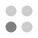• 9,875 Trophies Earned
• 485 Players Tracked
• 24 Total Trophies
• 176 Obtainable EXP
• 1,200 Points
• 380 Platinum Club
• 380 100% Club

### Pic-a-Pix Classic Trophies

•Collect all the trophies78.35%
•Solve a 5x5 puzzle100.00%
•Solve a 10x10 puzzle98.14%
•Solve a 10x10 puzzle without using an X97.53%
•Solve all the 10x10 puzzles88.04%
•Earn a medal for every 10x10 puzzle87.84%
•Solve a 15x15 puzzle91.34%
•Solve a 15x15 puzzle without using an X90.10%
•Solve all the 15x15 puzzles80.00%
•Earn a medal for every 15x15 puzzle80.00%
•Solve a 20x15 puzzle86.19%
•Solve a 20x15 puzzle without using an X86.19%
•Solve all the 20x15 puzzles79.18%
•Earn a medal for every 20x15 puzzle78.76%
•Solve a 20x20 puzzle84.54%
•Solve a 20x20 puzzle without using an X84.74%
•Solve all the 20x20 puzzles79.38%
•Earn a medal for every 20x20 puzzle78.76%
•Solve a 30x20 puzzle84.74%
•Solve a 30x20 puzzle without using an X84.12%
•Solve all the 30x20 puzzles80.41%
•Earn a medal for every 30x20 puzzle80.00%
•Solve every puzzle78.76%
•Earn a medal for every puzzle78.35%